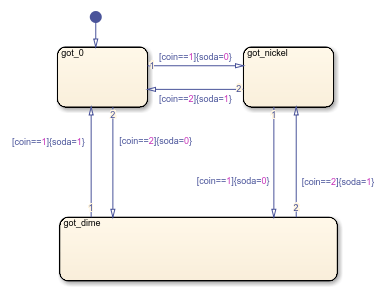Main Content

# Model a Vending Machine by Using Mealy Semantics

This example shows how to use Mealy semantics to model a vending machine.### Logic of the Mealy Vending Machine

In this example, the vending machine requires 15 cents to release a can of soda. The purchaser can insert a nickel or a dime, one at a time, to purchase the soda. The chart behaves like a Mealy machine because its output `soda` depends on both the input coin and current state:

When initial state `got_0` is active. No coin has been received or no coins are left.

• If a nickel is received (`coin == 1`), output `soda` remains 0, but state `got_nickel` becomes active.

• If a dime is received (`coin == 2`), output `soda` remains 0, but state `got_dime` becomes active.

• If input coin is not a dime or a nickel, state `got_0` stays active and no soda is released (output `soda = 0`).

In active state `got_nickel`. A nickel was received.

• If another nickel is received (`coin == 1`), state `got_dime` becomes active, but no can is released (`soda` remains at 0).

• If a dime is received (`coin == 2`), a can is released (`soda = 1`), the coins are banked, and the active state becomes `got_0` because no coins are left.

• If input coin is not a dime or a nickel, state `got_nickel` stays active and no can is released (output `soda = 0`).

In active state `got_dime`. A dime was received.

• If a nickel is received (`coin == 1`), a can is released (`soda = 1`), the coins are banked, and the active state becomes `got_0` because no coins are left.

• If a dime is received (`coin == 2`), a can is released (`soda = 1`), 15 cents are banked, and the active state becomes `got_nickel` because a nickel (change) is left.

• If input coin is not a dime or a nickel, state `got_dime` stays active and no can is released (output `soda = 0`).

### Design Rules in Mealy Vending Machine

This example of a Mealy vending machine illustrates these Mealy design rules:

• The chart computes outputs in condition actions.

• There are no state actions or transition actions.

• The chart defines chart inputs (`coin`) and outputs (`soda`).

• The value of the input `coin` determines the output: whether or not soda is released.

## SupportGet trial now